## Calculating ratios

Don't know how to use a machine? Looking for efficient setups? Stuck in a mission?
Premier2k
Burner Inserter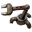Posts: 5
Joined: Thu May 30, 2019 6:59 am
Contact:

### Calculating ratios

Hey all,

After almost 300 hours in this game and 1 rocket launched I finally feel like I'm past the super newbie stage and now heading into 'fully addicted slight newbie' stage.
Previously (and in my current game) I'm not considering ratios or numbers and I'm just building what feels right. For example, to build something like yellow belts I'll just chuck down 6 assem. 2 machines and let it go to work. but now I'd really like to understand the process of all the calculations.

I've seen there are sites like kirk mcdonalds ratio calculator but 1. I have no idea how to use it and 2. I'd like to work this stuff out for myself.

So my questions I guess are, how do you go about starting to calculate? What are you calculating? Are you calculating for end game?
I see lots of comments about 10 science per minute or whatever and I'm wondering how you know that? How do you calculate how many green circuits to produce at any given time?

Can someone give me a guide on how to do this? or point me in the direction of a link. I've done some popular search site searching but have so far come up with nothing that's helped.

Treat me like an idiot and tell me all you know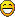Because as we all know, the factory must grow.

Thanks all,
Premier2k

Koub
Global Moderator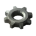Posts: 4787
Joined: Fri May 30, 2014 8:54 am
Contact:

### Re: Calculating ratios

[Koub] Moved to Gameplay Help.
What you're looking for is here : viewforum.php?f=134
My current personal favourite is https://kirkmcdonald.github.io/
You can also make all the calculations by yourself, but it's tedious, and automation is best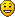Koub - Please consider English is not my native language.

zOldBulldog
Smart Inserter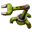Posts: 1103
Joined: Sat Mar 17, 2018 1:20 pm
Contact:

### Re: Calculating ratios

I found the Max Rate Calculator mod (https://mods.factorio.com/mod/MaxRateCalculator) to be the easiest way to move into calculating. There are even some YouTube videos about it.

jcranmer
Long Handed Inserter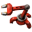Posts: 74
Joined: Wed Jun 29, 2016 9:59 pm
Contact:

### Re: Calculating ratios

If you want to understand how stuff like Kirk Mcdonald's calculator works, here's the actual math that goes down. (I've built a similar tool to his tool for personal use, so I have very good knowledge of the underlying details).

Let's start with a simple example. Suppose you want to make a red science pack. How many resources does it require? We can write down some equations:

1 red science = 1 iron gear + 1 copper plate
1 iron gear = 2 iron plate

With basic algebra, we can substitute the second equation in the first equation to get it in terms of our input resources:

1 red science = 2 iron plate + 1 copper plate

Green science looks more intimidating:
1 green science = 1 inserter + 1 yellow belt
1 inserter = 1 green circuit + 1 iron gear + 1 iron plate
2 yellow belt = 1 iron gear + 1 iron plate
1 green circuit = 3 copper cable + 1 iron plate
2 copper cable = 1 copper plate

Nevertheless, algorithmically, we can arrive at the answer by rewriting complex items in terms of simpler items and substituting as much as possible. There are lots of calculators out there for Factorio, and all of them will use this very basic core. Each recipe is an equation, and each item is a separate variable.

You'll note that I did the math here using units of resources. A lot of the use of calculators is for determining how many machines you need, which requires units of resource per time. To get those equations, we just divide the equation of a recipe by the crafting time of the recipe; since red science takes 5 seconds to craft, we arrive at an equation of ⅕ red science per second = ⅕ iron gear per second + ⅕ copper plate per second. Assemblers also have crafting time, which requires multiplying the equation by the crafting speed, and speed modules interact in the same way. Productivity modules require multiplying only one side of the equation--the outputs--by the productivity boost, which is not a linear effect and why they're so powerful.

If you step back a bit, what we're solving here is a set of linear equations, so we can apply linear algebra to the mix. We're trying to solve a matrix equation Ax = b, where b is a vector of the desired output in terms of resources per minute, x is a vector of the number of required factories, and A is a matrix which encodes all of the recipes. In the matrix A, each column corresponds to a recipe, each row an item, and each value is positive if the corresponding recipe produces that item, negative if it consumes it, or 0 if it's not directly involved. The recipe matrix is a sparse (nearly) triangular matrix, and the algorithm I discussed above is how you go about solving sparse triangular matrices.

There is one big wrinkle, though. I said that the recipe matrix is nearly triangular, and the "nearly" part matters in a big way. In vanilla Factorio, there are two violations of triangularity: oil and uranium. These violations means that we can't satisfy all desired output constraints: it's impossible to produce 10 heavy oil/second and no petroleum gas/second, for example. So we have to slacken our request for equality into an inequality; we want to solve for Ax ≥ b instead of Ax = b. It's now clear that we can have potentially infinite solutions to the inequalities, so we also want to pick the best solution, defined as minimizing c·x for some cost vector c. A reasonable cost vector for Factorio is to use the power consumption of each recipe; you can also minimize raw resource consumption with a balancing factor for the easy availability of water, but power consumption tends to automatically factor that in. This process is known as linear programming; it also adds in by default constraints that x≥0, but that's precisely what we want anyways (negative factories don't make sense).

So then, the most correct way to do the calculation is to create a linear program with the recipe matrix, desired final production rates, and cost vector, and then pass it to a tool capable of doing sparse linear programming. I will not get into the math of how solving linear programs works (it's very much in the category of "don't code this yourself"), but I will close with a useful tidbit. Solutions to linear programs must exist on one of the vertices of the polytope implied by the constraints. It turns out that the oil submatrix in vanilla Factorio produces 4 outputs (solid fuel, heavy oil, light oil, petroleum gas) for 8 recipes (3 refining recipes, 2 cracking, and 3 solid fuel recipes). Any vertex can only include 4 of these eight recipes. Considering that solid fuel consumption requires one of those recipes, and you need a refining recipe, that means you're either doing both cracking recipe (and converting only one oil type to solid fuel), or converting multiple things into solid fuel in lieu of cracking.

Premier2k
Burner InserterPosts: 5
Joined: Thu May 30, 2019 6:59 am
Contact:

### Re: Calculating ratios

Well that's an awesome response and explanation!

I've just been solving linear expressions and simplifying algebraic equations for my maths and algorithms module for my degree so I'm pretty familiar with all of that now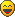As a developer, it might be an interesting side project to code my own calculator and see what figures I come up with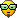Thanks for your response, it's given me much food for though.

Premier2k

### Who is online

Users browsing this forum: zaubara